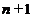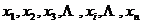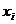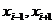Chapter 18           Optimization Methods

There are generally two types of optimization problems proposed in practical problems, one is to find the extreme value of the function, and the other is to find the extreme value of the functional. If the objective function ( function or functional ) has an obvious expression, it can generally be solved by analytical methods such as differential method, variational method, maximum ( small ) value principle or dynamic programming method ( indirect optimization ) . If the expression is too complex or even there is no obvious expression at all, it can be solved by numerical methods or direct methods such as "experimental optimization" ( direct optimization ) . The fifth chapter has introduced the method of finding the extreme value of the function by the differential method. This chapter introduces the direct method or experimental optimization method of the univariate and multivariate functions when the objective function has no obvious expression, and the unconditional and conditional extremum of the multivariate function. Numerical methods for value problems, variational methods for finding extreme values ​​of functional functions, maximum ( small ) value principle and dynamic programming ( dynamic programming methods can also be used to find extreme values ​​of ordinary functions ) .

Numerical or experimental optimization methods for finding the extrema of a function are sometimes called mathematical programming. In addition to linear programming, mathematical programming is collectively referred to as nonlinear programming. Mathematical programming generally deals with static problems, while variational method, maximum ( small ) value principle and dynamic programming generally deal with dynamic problems, but there is no clear boundary between the two.

§ 1   The solution to the extreme value problem of a single variable function ( direct method )

This section discusses finding the objective function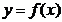The direct method ( or experimental optimization method ) of the optimal solution on the interval , since the minimum and maximum are only the difference of one sign of the objective function, so only the solution is discussed here.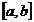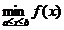The optimal solution of , that is to find a point on the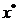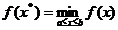At this time , itis called the optimal solution ( the optimal point ) .

[ Single peak function ]   If the function has only one extreme point on the interval ( thus it is the maximum point or the minimum point ) , then it is called a single peak function . The single peak function can also be defined by analytical methods as follows: Let it be the minimum point of the function on the interval , then there are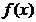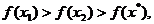when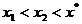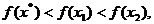when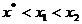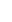It is also possible to define the case when it is the maximum point of the function on the interval .If the function has multiple extreme points in the interval , it is called a multimodal function . As long as the interval is properly divided , the function can be unimodal in each subinterval, so this section is limited to discussing unimodal functions.[ fraction method ]   by recurrence relation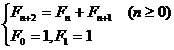The defined Fibonacci sequence produces a fractional sequence: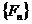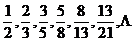If you want to limit the number of tests on the definition interval of the function to find the optimal point, you can divide the interval into equal parts, take the first test point at , and use the method of finding a symmetrical point ( symmetrical about the midpoint of the interval ) for subsequent test points ( Figure 18. 1) , a total of tests were done,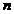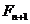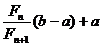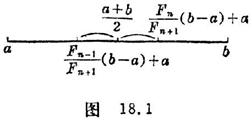can find the best point among the equal points in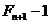Precision ( that is, the maximum possible distance between this best point and the actual minimum point

away ) is .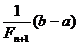The block diagram of the fraction method is as follows ( Figure 18.2 ) :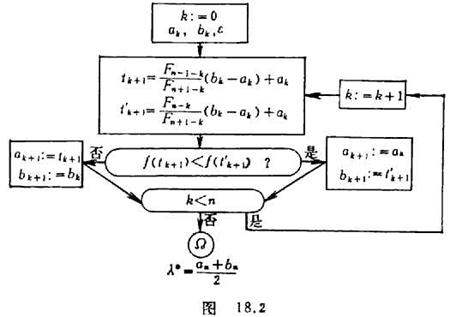where is the first search interval ( ) , and the minimum upper bound can be estimated by the following formula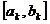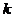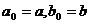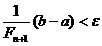where is the predetermined allowable error .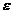The fractional method is the best way to limit the number of trials and only do one trial at a time .

[ 0.618 method ] can be proved in fraction method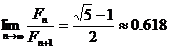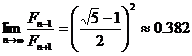Therefore, it is possible to approximate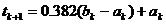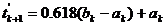The modified block diagram is as follows ( Figure 18.3 ) :

The selection of test points can also be calculated by the following formula: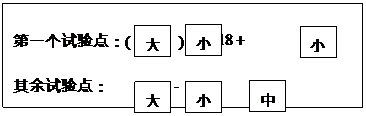middle

Note that here is the test point that has been done in the middle, not the midpoint. The method of shortening the search interval is the same as the fraction method.

The 0.618 method is also known as the golden section method, which is the optimal method for an unlimited number of batches and one test per batch .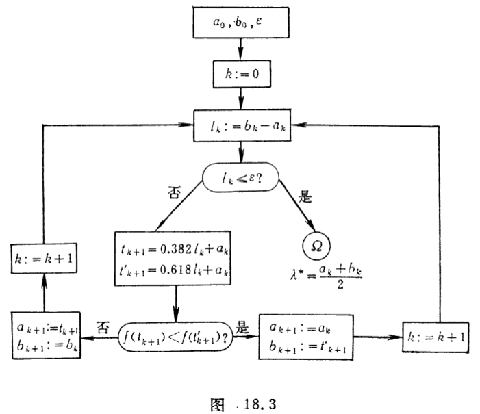[ Parabolic method ]   The test results set at three points are: Draw a quadratic parabola through three points on the plane ( Fig . 18.4 )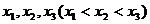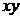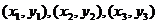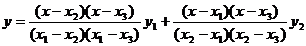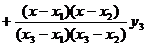Approximate the objective function , then use the minimum point of the parabola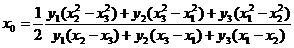The optimal point of the approximate objective function, the allowable error for a given objective function , if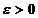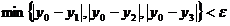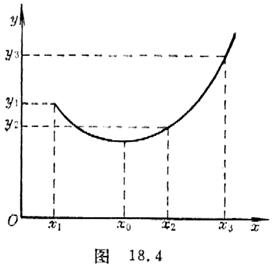Then take it as the approximate solution, otherwise, construct a new quadratic parabola with two points close to it, and approximate the optimal point with its minimum point.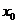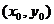This method works better when it is low in the middle and high at both ends, that is, when it is time .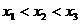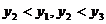If the and calculated by the above formula are equal, some modifications must be made .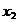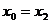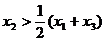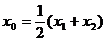[ Batch test method ]   There are several methods of batch test method according to the requirements, here only the average batch test method is introduced.

For example, to do a batch of tests ( positive integers ) , first divide the test range into equal parts, do each test at each point , and test and analyze the obtained results under the same conditions . If the best ( it is the function of this point) If the value is the smallest ) , keep the part ( ) , discard the rest, and then divide ( ) into equal parts, and then deal with it according to the above method .